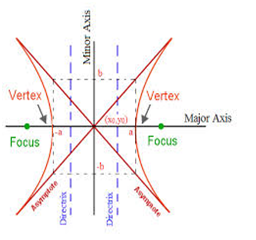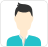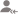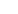truePost a Lesson

All

All

Lessons

Discussion

Lesson Posted on 18/08/2018 HyperbolaRaj Kumar

I am Six Sigma Black belt trained from American Society of Quality I am 2011 pass out in B.tech from...

The standard equation of hyperbola with reference to its principal axis along the coordinate axis is given by x2/a2 - y2/b2 = 1, where b2 = a2 (e2 -1) The foci of the hyperbola are S(ae, 0) and S’ = (-ae, 0) Equations of the directrices are given by x = a/e and x = -a/e The coordinates... read more

The standard equation of hyperbola  with reference to its principal axis along the coordinate axis is given by x2/a2 - y2/b2 = 1, where b2 = a2 (e2 -1)

The foci of the hyperbola are S(ae, 0) and S’ = (-ae, 0)

Equations of the directrices are given by x = a/e and x = -a/e

The coordinates of vertices are A’ = (-a, 0) and A = (a,0)

The length of latus rectum is 2b2/a = 2a(e2 - 1)

The length of the transverse axis of the hyperbola is 2a.

The difference of the focal distances of any point on the hyperbola is constant and is equal to transverse axis.

x2/a2 - y2/b2 = 1 and -x2/a2 + y2/b2 = 1 are conjugate hyperbola of each other.Dislike BookmarkSujoy D.

Tutor

x2 - y2 = a2 equation of rectangular hyperbola.
Dislike BookmarkSrinivas

Tutor.

y-k =+/- b/a( x-h).
Dislike BookmarkSujoy D.

Tutor

It is a line such that the distance between the curve and the line approaches zero as they tend to infinity.an asymptote of a curve is a line such that the distance between the curve and the line approaches zero as they tend to infinity.
Dislike BookmarkSujoy D.

Tutor

y= mx + sqrt(a*a*m*m - b*b)
Dislike BookmarkAnkit

JEE/Board Maths Tutor

(x-h)2/a2-(y-k)2/b2 = 1 2 means square.
Dislike BookmarkVikas K.

Physics and Mathematics Teacher

X^2/a^2 - Y^2/b^2 = 1, Where a and b are constants. Let me know if your question was for the equation of Normal to the parabola rather.
Dislike BookmarkAnkit

JEE/Board Maths Tutor

In geometry, two diameters of a conic section are said to be conjugate if each chord parallel to one diameter is bisected by the other diameter.
Dislike Bookmark

Prove that the midpoints of chords of the hyperbola (square of x)/(square of a)--(square of y)/(square... read more
Prove that the midpoints of chords of the hyperbola (square of x)/(square of a)–(square of y)/(square of b) = 1 parallel to the diameter y = mx lie on the diameter (square of a)my = (square of b) x? read lessAravind B.

Let the cord parallel to y=mx be y=mx+c..............(1) Then the points of intersection with the hyperbola are given by:- x^2/a^2 - (mx+c)^2/b^2 = 1 or (1/a^2-m^2/b^2)x^2-2mxc/b^2-c^2/b^2-1=0 .....(2) Let (x1,y1) and (x2,y2) be the end points of the cord. Then x1 and x2 both satisfy... read more
Let the cord parallel to y=mx be y=mx+c..............(1) Then the points of intersection with the hyperbola are given by:- x^2/a^2 - (mx+c)^2/b^2 = 1 or (1/a^2-m^2/b^2)x^2-2mxc/b^2-c^2/b^2-1=0 .....(2) Let (x1,y1) and (x2,y2) be the end points of the cord. Then x1 and x2 both satisfy Eq.(2) So x1+x2 = [2mc/b^2]/(1/a^2-m^2/b^2) = 2mc/(b^2/a^2-m^2)...............(3) Let (h,k) be the midpoint of the cord. Then h=(x1+x2)/2 = mc/(b^2/a^2-m^2) and k=(y1+y2)/2. Now both (x1,y1) and (x2,y2) satisfy Eq(1) so that k=mh+c. Substituting the value of c from Eq(3) k=mh+(b^2/a^2-m^2)h/m = b^2/(a^2m) Or a^2 mk = b^2h Therefore the locus of the midpoint (h,k) of the cord is a^2 my = b^2x. read less
Dislike Bookmark

Prove that the angle subtended by any chord of a rectangular hyperbola at the centre is the supplement... read more
Prove that the angle subtended by any chord of a rectangular hyperbola at the centre is the supplement of the angle between the tangents at the end of the chord? read lessMohit Sardana

Software Engineer

Solve with the help of parametric equations of hyperbola.
Dislike Bookmark

UrbanPro.com helps you to connect with the best in India. Post Your Requirement today and get connected.

Overview

Questions 25

Total Shares39 Followers
x

X

### Find Tutors, Trainers & Institutes near you

Post requirement and connect with the tutors in your locality

• Post a learning requirement
• Get customized responses
• Compare and select the best### Want to learn something New?

Find best tutors, trainers & institutes near you on UrbanProUrbanPro.com is India's largest network of most trusted tutors and institutes. Over 25 lakh students rely on UrbanPro.com, to fulfill their learning requirements across 1,000+ categories. Using UrbanPro.com, parents, and students can compare multiple Tutors and Institutes and choose the one that best suits their requirements. More than 6.5 lakh verified Tutors and Institutes are helping millions of students every day and growing their tutoring business on UrbanPro.com. Whether you are looking for a tutor to learn mathematics, a German language trainer to brush up your German language skills or an institute to upgrade your IT skills, we have got the best selection of Tutors and Training Institutes for you. Read more